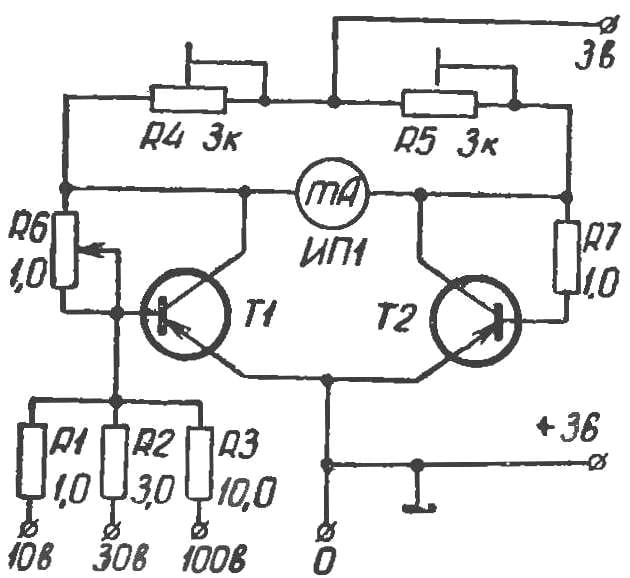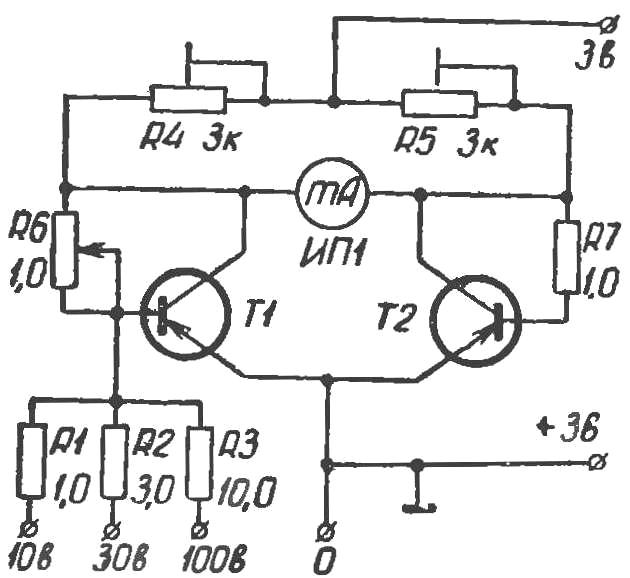# A simple voltmetermeasuring constant voltage value of 100 V. It is produced by the bridge circuit for the transistors T1 and T2. In one diagonal bridge included a measuring device in the other power source.

Adjustment of the voltmeter consists of two stages. First, changing the values of resistors R4 and R5, to achieve the equality of voltages at the collectors of transistors T1 and T2. Then use the variable resistor R6 set the arrow of the measuring device to zero.

Measured the voltage across the resistors R1, R2 and R3 is supplied to the base of transistor T1. This disturbed the balance of the bridge, and through the milliammeter begins to flow the current proportional to voltage.

Resistors R1 — R3 select with an accuracy of ±5%.

This scheme can be used as a prefix to the avometr with low input resistance.